# DAV Class 5 Maths Chapter 6 Worksheet 1 Solutions

The DAV Maths Book Class 5 Solutions and DAV Class 5 Maths Chapter 6 Worksheet 1 Solutions of Addition and Subtraction of Decimal Numbers offer comprehensive answers to textbook questions.

## DAV Class 5 Maths Ch 6 Worksheet 1 Solutions

Question 1.
(a)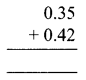Solution: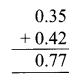(b)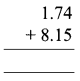Solution: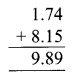(c)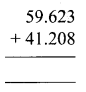Solution: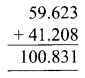(d)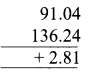Solution: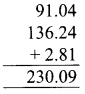(e)Solution:(f)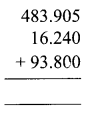Solution: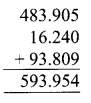Question 2.
(a) 0.35 + 0.62
Solution:
Convert into like decimals then add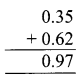(b) 8.496 + 2.564
Solution: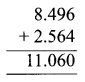(c) 25.08 + 5.8
Solution:(d) 14.9 + 64.941
Solution:(e) 3.19 + 27.974 + 8.8
Solution:(f) 31.001 + 13.01 + 131.1
Solution: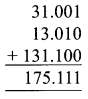(g) 7.8 + 31.856 + 0.956
Solution:(h) 191.38 + 12.904 + 76.1
Solution: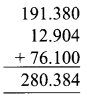DAV Class 5 Maths Chapter 6 Worksheet 1 Notes

The fractions in which the denominators are 10, 100, 1000, etc. are known as decimal numbers.
e.g. $$\frac{1}{10}$$ = .1, $$\frac{1}{100}$$ = .03, $$\frac{4}{1000}$$ = .004

Like decimals:
The decimal numbers which have the same number of decimal places are called like decimals
e.g. 3.26, 5.78.

Unlike decimals:
The decimal numbers have different numbers of decimal places, unlike decimals.
eg. 1.001, 3.280.We can convert unlike decimals into like decimals without changing their values. Adding zeroes to the right of a decimal number makes them like decimals.
e.g. 3.3, 4.73, 1.393 – Unlike decimals
3.300, 4.730, 1.393 – Like decimals

If we change the order of the addends, the sum remains the same.
e.g. 1.2 + 2.4 = 3.6 or 2.4 + 1.2 = 3.6

If we change the groupings of the addends then also the sum remains the same.
e.g. 2.3 + (1.4 + 3.3) = 2.3 + 4.7 = 7.0
or
(2.3 + 1.4) + 3.3 = 3.7 + 3.3 = 7.0

If zero is added to any decimal number or a decimal number is added to zero, the sum is the number itself.
e.g. 7.2 + 0 = 7.2
0 + 4.2 = 4.2

When we subtract zero from a decimal number, we get the decimal number itself.
e.g. 7.2 – 0 = 7.2

We can add decimal numbers in the same way as we add whole numbers.
e.g. Add like decimals 3.56 and 5.56 3 56

• Arrange the digits in the place value columns.
• The decimal point should come one below the other in the column.